Courses

# Worksheet Questions- Understanding Quadrilaterals Class 8 Notes | EduRev

## Class 8 : Worksheet Questions- Understanding Quadrilaterals Class 8 Notes | EduRev

The document Worksheet Questions- Understanding Quadrilaterals Class 8 Notes | EduRev is a part of the Class 8 Course Mathematics (Maths) Class 8.
All you need of Class 8 at this link: Class 8

Worksheet- 1

1. What is the sum of all the interior angles of a polygon having ‘n’ sides?
2. What is the sum of all the exterior angles of a polygon?
3. What is the measure of an exterior angle of a regular octagon?
4. What is the measure of an interior angle of a regular hexagon?
5. If the exterior angle of a regular polygon is 72°, then what is the number of sides of the polygon?
6. If the interior angle of a regular polygon is 144°, then what is the number of its sides?

1. (n – 2) x 180°
2. 360°
3. 45°
4. 120°
5. 5
6. 10

Worksheet- 21. In a parallelogram ABCD, if m∠A = 124°, then find the m∠B.
2. PQRS is a parallelogram such that m–R = 110°, then find m–P and –S.
3. Two opposite angles of a parallelogram are (5x – 8)° and (2x + 82)°. Find the measures of each angle of the parallelogram.
4. In the figure, find all the angles of the parallelogram ABCD, if m–A : m–B = 1 : 4.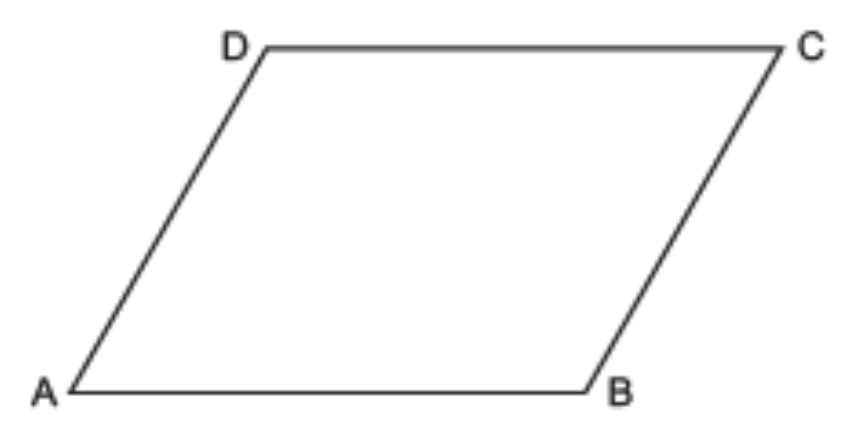5. Find the perimeter of the parallelogram BCDE.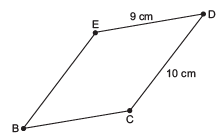6. In the figure, PQRS is a parallelogram. Find x, y and z.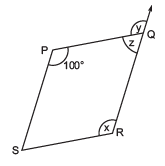7. JKLM is a parallelogram. If m–J = 70°, then find all other angles.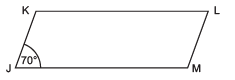8. In a parallelogram if measures of two adjacent angles are in the ratio of 4 : 5, find all the angles.
9. ABCD is a parallelogram. Given than OC = 4 cm and DB is 5 cm more than AC, find OD.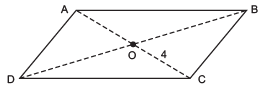10. If ABCD is an isosceles trapezium such that m–CDA = 3x and m–BAD = 2x. Find all angles of the trapezium.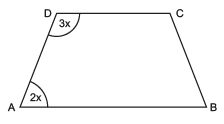2. m∠P = 110° and m∠S = 70°
3. 142°, 38°, 142°, 38°
4. 36°, 144°, 36°, 144°

5. 38 cm
6. x = 100°, y = 100°, z = 80°
7. m∠M = 110°, m∠L = 70° and m∠K = 110°.
8. 80°, 100°, 80°, 100°
9. 6.5 cm
10. 108°, 72°, 108°, 72°.

Worksheet- 31. Fill in the blanks:
(i) The diagonals of a rhombus bisect each other at ________.
(ii) All angles of a rectangle are equal and are ________ angles.
(iii) All sides of a ________ are equal and all angles are right angles.
(iv) A rhombus is a quadrilateral with ________ of equal length.
(v) A square has sides of equal length and angles of equal measure, so it is a ________ polygon.

2. Write ‘True’ or ‘False’ for each of the following:
(i) Every rhombus is a square.
(ii) Every square is a rhombus.
(iii) Every rhombus is a rectangle.
(iv) Every rectangle is a rhombus.
(v) Every rhombus is a parallelogram.
(vi) Diagonals of a rhombus need not be equal in length.
(vii) Every parallelogram is a rectangle.

(ii) right
(iii) square
(iv) sides
(v) regular
2. (i)  False
(ii) True
(iii) False
(iv) False
(v) True
(vi) True
(vii) False

Offer running on EduRev: Apply code STAYHOME200 to get INR 200 off on our premium plan EduRev Infinity!

## Mathematics (Maths) Class 8

135 videos|321 docs|48 tests

,

,

,

,

,

,

,

,

,

,

,

,

,

,

,

,

,

,

,

,

,

;